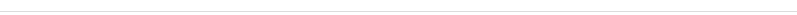# 声能

$W=W_{\mathrm {potential} }+W_{\mathrm {kinetic} }=\int _{V}{\frac {p^{2}}{2\rho _{0}c^{2}}}dV+\int _{V}{\frac {\rho _{0}v^{2}}{2}}dV$

• $$V$$ – 流体的体积
• $$p$$ – 声压
• $$v$$ – 流体质点速度
• $$\rho_0$$ – 流体静态密度
• $$c$$ – 声速

## 声能密度

$\epsilon ={\frac {p^{2}}{2\rho _{0}c^{2}}}+{\frac {\rho _{0}v^{2}}{2}}$

## 平均声能量密度

${\bar {\epsilon }}={\frac {p_{e}^{2}}{\rho _{0}c^{2}}}$

## 参考

1.  L. E. Kinsler, A. R. Frey, A. B. Coppens, and J. V. Sanders, 1999. Fundamentals of Acoustics, fourth edition (Wiley).第5.8节。
2. ^ 杜功焕、朱哲明、龚秀芳，声学基础，第二版，第4.7节。

## 电脑技术：KODI——家庭多媒体中心

KODI是一款多媒体操作系统，安装后就可以当作多媒体中心，可以看视频，听音乐，听流媒体广播，甚至看一些特殊频道，都是可以的。## 电脑技术：软路由搭建攻略——从小白到大白[转载]

• 软路由的定义
• 软路由的优缺点
• 什么人会使用软路由
• 软路由的挑选
• 软路由的组建
• 软路由的设置
• 硬路由桥接软路由发射WiFi

这篇文章不会涉及的知识点：

• 软路由虚拟机多系统（对于新手来说太麻烦而且我个人觉得暂时没有必要）
• 黑群晖之类的NAS搭建（三大妈已经有其他作者写过啦）

总的来说就是作者会尽可能的从新手的角度出发，尽量让各位小白都能看懂然后早日打造出自己的软路由什么是软路由

在了解什么是软路由之前，我们先定义一下【硬路由】这个关键词，硬路由就是我们现在随便在京东淘宝搜索【路由器】搜出来的，从一开始就是按照路由器规范设计出来的硬件设备。而相对的【软路由】则是指，利用现有的硬件（可能是电脑或者树莓派之类的）配合软件来实现路由器的功能。

软路由对比硬路由优势在哪里？

## Spotmini## Laikago## Anymal